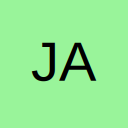# My function needs to know what cell, row and column it is in?

• Hi,

I am looking for some VBA that will tell my function what cell it is in, for example:

Code
``````function wibble()

wibble = ("I am in cell " & X & "or column " & 1 & " and row " & 2)

End function``````

The output would be: 1 am in cell A1 or column 1 and row 1

On an Excel worksheet the equivalent is "=ROW()" or "=COLUMN()" but there does not appear to be an application.worksheetfunction equivalent.

Many thanks

James

• Re: My function needs to know what cell, row and column it is in?

Can only assume you want the address of the active cell

Code
``````MsgBox "I am in cell " & ActiveCell.Address

MsgBox "I am in Column " &  ActiveCell.Column & " and row " & ActiveCell.Row``````
• Re: My function needs to know what cell, row and column it is in?

Code
``````Function wibble()

If TypeOf Application.Caller Is Range Then
wibble = "I am in cell " & Application.Caller.Address(0, 0)
End If

End Function``````

?

• Re: My function needs to know what cell, row and column it is in?

Many thanks!

## Participate now!

Don’t have an account yet? Register yourself now and be a part of our community!